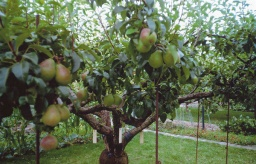# Section 6538

The tourist section planted 145 trees in the spring, which is 15% more than planned. How many trees did they plan to grow?

x =  126

### Step-by-step explanation:

145 = x + 15/100 x

145 = x + 15/100·x

115x = 14500

x = 14500/115 = 126.08695652

x = 2900/23 ≈ 126.086957

Our simple equation calculator calculates it.Did you find an error or inaccuracy? Feel free to write us. Thank you!

Tips for related online calculators